# Positive solutions to second-order differential equations with dependence on the first-order derivative and nonlocal boundary conditions

## Abstract

In this paper, we consider the existence of positive solutions for second-order differential equations with deviating arguments and nonlocal boundary conditions. By the fixed point theorem due to Avery and Peterson, we provide sufficient conditions under which such boundary value problems have at least three positive solutions. We discuss our problem both for delayed and advanced arguments α and also in the case when $\alpha \left(t\right)=t$, $t\in \left[0,1\right]$. In all cases, the argument β can change the character on $\left[0,1\right]$, see problem (1). It means that β can be delayed in some set $\overline{J}\subset \left[0,1\right]$ and advanced in $\left[0,1\right]\setminus \overline{J}$. An example is added to illustrate the results.

MSC:34B10.

## 1 Introduction

Put $J=\left[0,1\right]$, ${\mathbb{R}}_{+}=\left[0,\mathrm{\infty }\right)$. Let us consider the following boundary value problem:

$\left\{\begin{array}{c}{x}^{″}\left(t\right)+h\left(t\right)f\left(t,x\left(\alpha \left(t\right)\right),{x}^{\prime }\left(\beta \left(t\right)\right)\right)=0,\phantom{\rule{1em}{0ex}}t\in \left(0,1\right),\hfill \\ x\left(0\right)=\gamma x\left(\eta \right)+{\lambda }_{1}\left[x\right],\phantom{\rule{2em}{0ex}}x\left(1\right)=\xi x\left(\eta \right)+{\lambda }_{2}\left[x\right],\phantom{\rule{1em}{0ex}}\eta \in \left(0,1\right),\hfill \end{array}$
(1)

where ${\lambda }_{1}$, ${\lambda }_{2}$ denote linear functionals on $C\left(J\right)$ given by

${\lambda }_{1}\left[x\right]={\int }_{0}^{1}x\left(t\right)\phantom{\rule{0.2em}{0ex}}dA\left(t\right),\phantom{\rule{2em}{0ex}}{\lambda }_{2}\left[x\right]={\int }_{0}^{1}x\left(t\right)\phantom{\rule{0.2em}{0ex}}dB\left(t\right)$

involving Stieltjes integrals with suitable functions A and B of bounded variation on J. It is not assumed that ${\lambda }_{1}$, ${\lambda }_{2}$ are positive to all positive x. As we see later, the measures dA, dB can be signed measures.

We introduce the following assumptions:

H1: $f\in C\left(J×{\mathbb{R}}_{+}×\mathbb{R},{\mathbb{R}}_{+}\right)$, $\alpha ,\beta \in C\left(J,J\right)$, A and B are functions of bounded variation;

H2: $h\in C\left(J,{\mathbb{R}}_{+}\right)$ and h does not vanish identically on any subinterval;

H3: $1-\gamma -{\lambda }_{1}\left[p\right]>0$ or $1-\xi -{\lambda }_{2}\left[p\right]>0$ for $p\left(t\right)=1$, $t\in J$, $\gamma ,\xi \ge 0$.

Recently, the existence of multiple positive solutions for differential equations has been studied extensively; for details, see, for example, . However, many works about positive solutions have been done under the assumption that the first-order derivative is not involved explicitly in nonlinear terms; see, for example, [3, 6, 814, 17, 20, 2527, 30]. From this list, only papers [912, 14, 20, 30] concern positive solutions to problems with deviating arguments. On the other hand, there are some papers considering the multiplicity of positive solutions with dependence on the first-order derivative; see, for example, [2, 4, 5, 7, 15, 16, 18, 19, 2124, 28, 29, 31]. Note that boundary conditions (BCs) in differential problems have important influence on the existence of the results obtained. In this paper, we consider problem (1) which is a problem with dependence on the first-order derivative with BCs involving Stieltjes integrals with signed measures of dA, dB appearing in functionals ${\lambda }_{1}$, ${\lambda }_{2}$; moreover, problem (1) depends on deviating arguments.

For example, in papers [2, 4, 15, 18, 22, 24], the existence of positive solutions to second-order differential equations with dependence on the first-order derivative (but without deviating arguments) has been studied with various BCs including the following: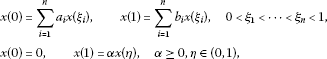by fixed point theorems in a cone (such as Avery-Peterson, an extension of Krasnoselskii’s fixed point theorem or monotone iterative method) with corresponding assumptions:

${a}_{i},{b}_{i}\in \left(0,1\right),i=1,2,\dots ,n,\phantom{\rule{1em}{0ex}}\sum _{i=1}^{n}{a}_{i},\sum _{i=1}^{n}{b}_{i}\in \left(0,1\right),$

or $1-\alpha \eta >0$, respectively.

For example, in papers [811, 20, 22, 30], the existence of positive solutions to second-order differential equations including impulsive problems, but without dependence on the first-order derivative, has been studied with various BCs including the following: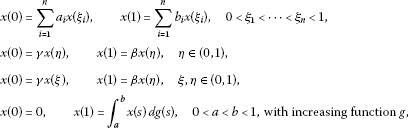under corresponding assumptions by fixed point theorems in a cone (such as Avery-Peterson, Leggett-Williams, Krasnoselskii or fixed point index theorem). See also paper , where positive solutions have been discussed for second-order impulsive problems with boundary conditions

$x\left(0\right)=0,\phantom{\rule{2em}{0ex}}x\left(1\right)={\int }_{0}^{1}x\left(s\right)\phantom{\rule{0.2em}{0ex}}dA\left(s\right);$

here ${\lambda }_{1}$ has the same form as in problem (1) with signed measure dA appearing in functional ${\lambda }_{1}$.

Positive solutions to second-order differential equations with boundary conditions that involve Stieltjes integrals have been studied in the case of signed measures in papers [25, 26] with BCs including, for example, the following:The main results of papers [25, 26] have been obtained by the fixed point index theory for problems without deviating arguments. The study of positive solutions to boundary value problems with Stieltjes integrals in the case of signed measures has also been done in papers [3, 7, 13, 14, 27] for second-order differential equations (also impulsive) or third-order differential equations by using the fixed point index theory, the Avery-Peterson fixed point theorem or fixed point index theory involving eigenvalues.

Note that BCs in problem (1) with functionals ${\lambda }_{1}$, ${\lambda }_{2}$ cover some nonlocal BCs, for example,for some constants ${a}_{i}$, ${b}_{i}$ and some functions ${g}_{1}$, ${g}_{2}$. In our paper, the assumption that the measures dA, dB in the definitions of ${\lambda }_{1}$, ${\lambda }_{2}$ are positive is not needed. More precisely, one needs to choose the above functions ${g}_{1}$, ${g}_{2}$ in such a way that the assumption H4 holds. It means that ${g}_{1}$, ${g}_{2}$ can change sign on J.

A standard approach (see, for example, ) to studying positive solutions of boundary value problems such as (1) is to translate problem (1) to a Hammerstein integral equation

$\begin{array}{rcl}x\left(t\right)& =& {\mathrm{\Gamma }}_{1}\left(t\right){\lambda }_{1}\left[x\right]+{\mathrm{\Gamma }}_{2}\left(t\right){\lambda }_{2}\left[x\right]+{\mathrm{\Gamma }}_{3}\left(t\right){\int }_{0}^{1}G\left(\eta ,s\right)h\left(s\right)f\left(s,x\left(\alpha \left(s\right)\right),{x}^{\prime }\left(\beta \left(s\right)\right)\right)\phantom{\rule{0.2em}{0ex}}ds\\ +{\int }_{0}^{1}G\left(t,s\right)h\left(s\right)f\left(s,x\left(\alpha \left(s\right)\right),{x}^{\prime }\left(\beta \left(s\right)\right)\right)\phantom{\rule{0.2em}{0ex}}ds\equiv \mathcal{W}x\left(t\right)\end{array}$
(2)

to find a solution as a fixed point of the operator $\mathcal{W}$ by using a fixed point theorem in a cone. ${\mathrm{\Gamma }}_{1}$, ${\mathrm{\Gamma }}_{2}$, ${\mathrm{\Gamma }}_{3}$ are corresponding continuous functions while ${\lambda }_{1}$ and ${\lambda }_{2}$ have the same form as in problem (1). G denotes a Green function connected with our problem, so in our case it is given by

$G\left(t,s\right)=\left\{\begin{array}{cc}s\left(1-t\right),\hfill & 0\le s\le t,\hfill \\ t\left(1-s\right),\hfill & t\le s\le 1.\hfill \end{array}$

In our paper, we eliminate ${\lambda }_{1}$ and ${\lambda }_{2}$ from problem (2) to obtain the equation $x=\overline{\mathcal{W}}x$ with a corresponding operator $\overline{\mathcal{W}}$, and then we seek solutions as fixed points of this operator $\overline{\mathcal{W}}$.

Note that if we put $\gamma =\xi =0$ in the BCs of problem (1), then this new problem is more general than the previous one because in this case someone, for example, can take ${\lambda }_{1}\left[x\right]=\gamma x\left(\eta \right)$, ${\lambda }_{2}\left[x\right]=\xi x\left(\eta \right)$. In this paper, we try to explain why for some cases we have to discuss problem (1) with constants $\gamma >0$ or $\xi >0$.

To apply such a fixed point theorem in a cone to problem (1), we have to construct a suitable cone K. Usually, we need to find a nonnegative function κ and a constant $\overline{\rho }\in \left(0,1\right]$ such that $G\left(t,s\right)\le \kappa \left(s\right)$ for $t,s\in J$ and $G\left(t,s\right)\ge \overline{\rho }\kappa \left(t\right)$ for $t\in \left[\eta ,\overline{\eta }\right]\subset \left[0,1\right]$ and $s\in J$ (see, for example, ) to work with the inequality

$\underset{\left[\eta ,\overline{\eta }\right]}{min}|x\left(t\right)|\ge \overline{\rho }\underset{t\in J}{max}|x\left(t\right)|.$

Indeed, for problems without deviating arguments, someone can use any interval $\left[\eta ,\overline{\eta }\right]\subset \left[0,1\right]$. It means that when $\alpha \left(t\right)=t$ on J, then we can take $\gamma =\xi =0$ in the boundary conditions of problem (1) to work with the inequality

$\underset{\left[\zeta ,\varrho \right]}{min}|x\left(t\right)|\ge \kappa \underset{t\in J}{max}|x\left(t\right)|$

for ζ, ϱ such that $\zeta +\varrho <1$, $0<\zeta <\varrho <1$ with $\kappa =min\left(\zeta ,1-\varrho \right)$; see Section 5.

Note that for problems with delayed or advanced arguments, we have to use interval $\left[0,\eta \right]\subset \left[0,1\right)$ or $\left[\eta ,1\right]\subset \left(0,1\right]$, respectively. We see that if $\gamma =\xi =0$, then $\overline{\rho }=0$ for problem (1) with deviated arguments. It shows that the approach from papers  needs a little modification to problems with delayed or advanced arguments. Consider the situation $\alpha \left(t\right)\le t$ on J. In this case, we can put $\xi =0$ in the boundary conditions of problem (1) to find a constant $\rho \in \left(0,1\right)$ to work with the inequality

$\underset{\left[0,\eta \right]}{min}|x\left(t\right)|\ge \rho \underset{t\in J}{max}|x\left(t\right)|;$

see Section 3. For the case $\alpha \left(t\right)\ge t$ on J, we can put $\gamma =0$ to work similarly as in Section 3; see Section 4. Note that in the above three cases for the argument β, we need only the assumption $\beta \in C\left(J,J\right)$, which means that β can change the character in J.

Note that in cited papers, positive solutions to differential equations with dependence on the first-order derivative have been investigated only for problems without deviating arguments, see [2, 4, 5, 7, 15, 16, 18, 19, 2124, 28, 29, 31]. Moreover, BCs in problem (1) cover some nonlocal BCs discussed earlier.

Motivated by , in this paper, we apply the fixed point theorem due to Avery-Peterson to obtain sufficient conditions for the existence of multiple positive solutions to problems of type (1). In problem (1), an unknown x depends on deviating arguments which can be both of advanced or delayed type. To the author’s knowledge, it is the first paper when positive solutions have been investigated for such general boundary value problems with functionals ${\lambda }_{1}$, ${\lambda }_{2}$ and with deviating arguments α, β in differential equations in which f depends also on the first-order derivative. It is important to indicate that problems of type (1) have been discussed with signed measures of dA, dB appearing in Stieltjes integrals of functionals ${\lambda }_{1}$, ${\lambda }_{2}$.

The organization of this paper is as follows. In Section 2, we present some necessary lemmas connected with our main results. In Section 3, we first present some definitions and a theorem of Avery and Peterson which is useful in our research. Also in Section 3, we discuss the existence of multiple positive solutions to problems with delayed argument α, by using the above mentioned Avery-Peterson theorem. At the end of this section, an example is added to verify theoretical results. In Section 4, we formulate sufficient conditions under which problems with advanced argument α have positive solutions. In the last section, we discuss problems of type (1) when $\alpha \left(t\right)=t$ on J.

## 2 Some lemmas

Let us introduce the following notations:

Lemma 1 Let $x\in {C}^{1}\left(J,\mathbb{R}\right)$, $p\left(t\right)=1$, $t\in J$. Assume that A and B are functions of bounded variation and, moreover,

$x\left(0\right)=\gamma x\left(\eta \right)+{\lambda }_{1}\left[x\right],\phantom{\rule{2em}{0ex}}x\left(1\right)=\xi x\left(\eta \right)+{\lambda }_{2}\left[x\right],\phantom{\rule{1em}{0ex}}\gamma ,\xi \ge 0,\eta \in \left(0,1\right)$

with

1. (i)

$1-\gamma -{\lambda }_{1}\left[p\right]\ne 0$ or

2. (ii)

$1-\xi -{\lambda }_{2}\left[p\right]\ne 0$.

Then

Here, VarA denotes the variation of a function A on J.

Proof Note that in case (i), we have

$\begin{array}{rcl}x\left(0\right)& =& \gamma x\left(\eta \right)+{\lambda }_{1}\left[x\right]\\ =& \gamma \left[x\left(\eta \right)-x\left(0\right)\right]+\gamma x\left(0\right)+{\int }_{0}^{1}\left(x\left(t\right)-x\left(0\right)\right)\phantom{\rule{0.2em}{0ex}}dA\left(t\right)+{\lambda }_{1}\left[p\right]x\left(0\right)\\ =& \gamma {\int }_{0}^{\eta }{x}^{\prime }\left(s\right)\phantom{\rule{0.2em}{0ex}}ds+{\int }_{0}^{1}\left({\int }_{0}^{t}{x}^{\prime }\left(s\right)\phantom{\rule{0.2em}{0ex}}ds\right)\phantom{\rule{0.2em}{0ex}}dA\left(t\right)+\gamma x\left(0\right)+{\lambda }_{1}\left[p\right]x\left(0\right),\end{array}$

so

$x\left(0\right)=\frac{1}{1-\gamma -{\lambda }_{1}\left[p\right]}\left[\gamma {\int }_{0}^{\eta }{x}^{\prime }\left(s\right)\phantom{\rule{0.2em}{0ex}}ds+{\int }_{0}^{1}\left({\int }_{0}^{t}{x}^{\prime }\left(s\right)\phantom{\rule{0.2em}{0ex}}ds\right)\phantom{\rule{0.2em}{0ex}}dA\left(t\right)\right].$

Hence,

$|x\left(0\right)|\le \frac{1}{|1-\gamma -{\lambda }_{1}\left[p\right]|}\left(\gamma +VarA\right){\parallel {x}^{\prime }\parallel }_{1}.$

Combining this with the relation

$x\left(t\right)=x\left(0\right)+{\int }_{0}^{t}{x}^{\prime }\left(s\right)\phantom{\rule{0.2em}{0ex}}ds,$

we obtain

${\parallel x\parallel }_{1}\le |x\left(0\right)|+{\parallel {x}^{\prime }\parallel }_{1}\le M{\parallel {x}^{\prime }\parallel }_{1}.$

This proves case (i).

In case (ii), similarly,

$\begin{array}{rcl}x\left(1\right)& =& \xi x\left(\eta \right)+{\lambda }_{2}\left[x\right]=\xi \left[x\left(\eta \right)-x\left(1\right)\right]+\xi x\left(1\right)-{\int }_{0}^{1}\left(x\left(1\right)-x\left(t\right)\right)\phantom{\rule{0.2em}{0ex}}dB\left(t\right)+{\lambda }_{2}\left[p\right]x\left(1\right)\\ =& -\xi {\int }_{\eta }^{1}{x}^{\prime }\left(s\right)\phantom{\rule{0.2em}{0ex}}ds-{\int }_{0}^{1}\left({\int }_{t}^{1}{x}^{\prime }\left(s\right)\phantom{\rule{0.2em}{0ex}}ds\right)\phantom{\rule{0.2em}{0ex}}dB\left(t\right)+\xi x\left(1\right)+{\lambda }_{2}\left[p\right]x\left(1\right),\end{array}$

so

$x\left(1\right)=-\frac{1}{1-\xi -{\lambda }_{2}\left[p\right]}\left[\xi {\int }_{\eta }^{1}{x}^{\prime }\left(s\right)\phantom{\rule{0.2em}{0ex}}ds+{\int }_{0}^{1}\left({\int }_{0}^{1}{x}^{\prime }\left(s\right)\phantom{\rule{0.2em}{0ex}}ds\right)\phantom{\rule{0.2em}{0ex}}dB\left(t\right)\right].$

Hence,

$|x\left(1\right)|\le \frac{1}{|1-\xi -{\lambda }_{2}\left[p\right]|}\left(\xi +VarB\right){\parallel {x}^{\prime }\parallel }_{1}.$

$x\left(t\right)=x\left(1\right)-{\int }_{t}^{1}{x}^{\prime }\left(s\right)\phantom{\rule{0.2em}{0ex}}ds,$

we get the result in case (ii). This ends the proof. □

Remark 1 If we assume that A and B are increasing functions, then there exists $\sigma \in J$ such that

$\begin{array}{rcl}x\left(0\right)& =& \frac{1}{1-\gamma -{\lambda }_{1}\left[p\right]}\left[\gamma {\int }_{0}^{\eta }{x}^{\prime }\left(s\right)\phantom{\rule{0.2em}{0ex}}ds+{\int }_{0}^{1}\left({\int }_{0}^{t}{x}^{\prime }\left(s\right)\phantom{\rule{0.2em}{0ex}}ds\right)\phantom{\rule{0.2em}{0ex}}dA\left(t\right)\right]\\ =& \frac{1}{1-\gamma -{\lambda }_{1}\left[p\right]}\left[\gamma {\int }_{0}^{\eta }{x}^{\prime }\left(s\right)\phantom{\rule{0.2em}{0ex}}ds+{\int }_{0}^{\sigma }{x}^{\prime }\left(s\right)\phantom{\rule{0.2em}{0ex}}ds{\int }_{0}^{1}\phantom{\rule{0.2em}{0ex}}dA\left(t\right)\right].\end{array}$

Hence,

$|x\left(0\right)|\le \frac{1}{|1-\gamma -{\lambda }_{1}\left[p\right]|}\left(\gamma +|{\int }_{0}^{1}\phantom{\rule{0.2em}{0ex}}dA\left(t\right)|\right){\parallel {x}^{\prime }\parallel }_{1}.$

Similarly, we can show that

$|x\left(1\right)|\le \frac{1}{|1-\xi -{\lambda }_{2}\left[p\right]|}\left(\xi +|{\int }_{0}^{1}\phantom{\rule{0.2em}{0ex}}dB\left(t\right)|\right){\parallel {x}^{\prime }\parallel }_{1}.$

Now, the constant M from Lemma 1 has the form

$M=1+\left\{\begin{array}{cc}\frac{1}{|1-\gamma -{\lambda }_{1}\left[p\right]|}\left(\gamma +|{\int }_{0}^{1}\phantom{\rule{0.2em}{0ex}}dA\left(t\right)|\right),\hfill & \text{in case (i)},\hfill \\ \frac{1}{|1-\xi -{\lambda }_{2}\left[p\right]|}\left(\xi +|{\int }_{0}^{1}\phantom{\rule{0.2em}{0ex}}dB\left(t\right)|\right),\hfill & \text{in case (ii)}.\hfill \end{array}$

Consider the following problem:

$\left\{\begin{array}{c}{u}^{″}\left(t\right)+y\left(t\right)=0,\phantom{\rule{1em}{0ex}}t\in \left(0,1\right),\hfill \\ u\left(0\right)=\gamma u\left(\eta \right)+{\lambda }_{1}\left[u\right],\phantom{\rule{2em}{0ex}}u\left(1\right)=\xi u\left(\eta \right)+{\lambda }_{2}\left[u\right],\phantom{\rule{1em}{0ex}}\eta \in \left(0,1\right),\gamma ,\xi \ge 0.\hfill \end{array}$
(3)

Let us introduce the assumption.

H0: A and B are functions of bounded variation and

$\begin{array}{rcl}\delta & \equiv & 1-\gamma +\eta \left(\gamma -\xi \right)\ne 0,\\ \mathrm{\Delta }& \equiv & {A}_{1}\left({B}_{2}-1+\xi \eta \right)+{A}_{2}\left(1-\xi -{B}_{1}\right)+\delta -\eta \gamma {B}_{1}-\left(1-\gamma \right){B}_{2}\ne 0\end{array}$

forWe require the following result.

Lemma 2 Let the assumption H0 hold and let $y\in {L}^{1}\left(J,\mathbb{R}\right)$. Then problem (3) has a unique solution given by

$\begin{array}{rcl}u\left(t\right)& =& \frac{1}{\mathrm{\Delta }}\left[1-\xi \eta -{B}_{2}-\left(1-\xi -{B}_{1}\right)t\right]{\lambda }_{1}\left[\overline{F}y\right]\\ +\frac{1}{\mathrm{\Delta }}\left[\eta \gamma +{A}_{2}+\left(1-\gamma -{A}_{1}\right)t\right]{\lambda }_{2}\left[\overline{F}y\right]+\overline{F}y\left(t\right)\end{array}$

with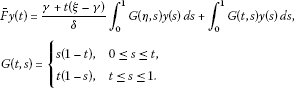Proof Integrating the differential equation in (3) two times, we have

$u\left(t\right)=u\left(0\right)+t{u}^{\prime }\left(0\right)-{\int }_{0}^{t}\left(t-s\right)y\left(s\right)\phantom{\rule{0.2em}{0ex}}ds.$
(4)

Put $t=1$ and use the boundary conditions from problem (3) to obtain

$\xi u\left(\eta \right)+{\lambda }_{2}\left[u\right]=\gamma u\left(\eta \right)+{\lambda }_{1}\left[u\right]+{u}^{\prime }\left(0\right)-{\int }_{0}^{1}\left(1-s\right)y\left(s\right)\phantom{\rule{0.2em}{0ex}}ds.$

Now, finding from this ${u}^{\prime }\left(0\right)$ and then substituting it to formula (4), we have

$u\left(t\right)=\left[\gamma +t\left(\xi -\gamma \right)\right]u\left(\eta \right)+\left(1-t\right){\lambda }_{1}\left[u\right]+t{\lambda }_{2}\left[u\right]+{\int }_{0}^{1}G\left(t,s\right)y\left(s\right)\phantom{\rule{0.2em}{0ex}}ds.$
(5)

Next, putting $t=\eta$, we can find $u\left(\eta \right)$, and then substitute it to formula (5) to obtain

$u\left(t\right)=\frac{1}{\delta }\left(\left[1-\xi \eta -\left(1-\xi \right)t\right]{\lambda }_{1}\left[u\right]+\left[\eta \gamma +\left(1-\gamma \right)t\right]{\lambda }_{2}\left[u\right]\right)+\overline{F}y\left(t\right).$
(6)

Now, we have to eliminate ${\lambda }_{1}\left[u\right]$ and ${\lambda }_{2}\left[u\right]$ from (6). If u is a solution of (6), then

$\left\{\begin{array}{c}{\lambda }_{1}\left[u\right]=\frac{1}{\delta }\left[\left(1-\xi \eta \right){A}_{1}-\left(1-\xi \right){A}_{2}\right]{\lambda }_{1}\left[u\right]+\frac{1}{\delta }\left[\eta \gamma {A}_{1}+\left(1-\gamma \right){A}_{2}\right]{\lambda }_{2}\left[u\right]+{\lambda }_{1}\left[\overline{F}y\right],\hfill \\ {\lambda }_{2}\left[u\right]=\frac{1}{\delta }\left[\left(1-\xi \eta \right){B}_{1}-\left(1-\xi \right){B}_{2}\right]{\lambda }_{1}\left[u\right]+\frac{1}{\delta }\left[\eta \gamma {B}_{1}+\left(1-\gamma \right){B}_{2}\right]{\lambda }_{2}\left[u\right]+{\lambda }_{2}\left[\overline{F}y\right].\hfill \end{array}$

Solving this system with respect to ${\lambda }_{1}\left[u\right]$, ${\lambda }_{2}\left[u\right]$ and then substituting to (6), we have the assertion of this lemma. This ends the proof. □

Define the operator T by

$\begin{array}{rcl}Tu\left(t\right)& =& \frac{1}{\mathrm{\Delta }}\left[1-\xi \eta -{B}_{2}-\left(1-\xi -{B}_{1}\right)t\right]{\lambda }_{1}\left[Fu\right]\\ +\frac{1}{\mathrm{\Delta }}\left[\eta \gamma +{A}_{2}+\left(1-\gamma -{A}_{1}\right)t\right]{\lambda }_{2}\left[Fu\right]+Fu\left(t\right)\end{array}$

with

$\begin{array}{rcl}Fu\left(t\right)& =& \frac{\gamma +t\left(\xi -\gamma \right)}{\delta }{\int }_{0}^{1}G\left(\eta ,s\right)h\left(s\right)f\left(s,u\left(\alpha \left(s\right)\right),{u}^{\prime }\left(\beta \left(s\right)\right)\right)\phantom{\rule{0.2em}{0ex}}ds\\ +{\int }_{0}^{1}G\left(t,s\right)h\left(s\right)f\left(s,u\left(\alpha \left(s\right)\right),{u}^{\prime }\left(\beta \left(s\right)\right)\right)\phantom{\rule{0.2em}{0ex}}ds.\end{array}$

We consider the Banach space $E=\left({C}^{1}\left(J,\mathbb{R}\right),\parallel \cdot \parallel \right)$ with the maximum norm $\parallel x\parallel =max\left({\parallel x\parallel }_{1},{\parallel {x}^{\prime }\parallel }_{1}\right)$. Define the cone $K\subset E$ by

$K=\left\{x\in E:x\left(t\right)\ge 0,t\in J,{\lambda }_{1}\left[x\right]\ge 0,{\lambda }_{2}\left[x\right]\ge 0,\underset{\left[0,\eta \right]}{min}x\left(t\right)\ge \rho {\parallel x\parallel }_{1}\right\}$

with

$\rho =min\left(\gamma \left(1-\eta \right),1-\eta ,\frac{\eta \gamma }{1+\gamma \left(\eta -1\right)}\right),\phantom{\rule{1em}{0ex}}\gamma >0.$

Let us introduce the following assumption.

H4: A and B are functions of bounded variation and

1. (i)

$\delta >0$, $\mathrm{\Delta }>0$, ${A}_{j}\ge 0$, ${B}_{j}\ge 0$, ${\mathcal{G}}_{j}\left(s\right)\ge 0$ for $j=1,2$ where ${A}_{j}$, ${B}_{j}$, ${\mathcal{G}}_{j}$, δ, Δ are defined as in the assumption H0,

2. (ii)

$\gamma \left({A}_{1}-{A}_{2}\right)+\xi {A}_{2}\ge 0$, $\gamma \left({B}_{1}-{B}_{2}\right)+\xi {B}_{2}\ge 0$, $\eta \gamma {B}_{1}+\left(1-\gamma \right){B}_{2}\ge 0$, $\left(1-\xi \eta \right){A}_{1}-\left(1-\xi \right){A}_{2}\ge 0$, ${B}_{1}-{B}_{2}\ge 0$, $\delta -\eta \gamma {B}_{1}-\left(1-\gamma \right){B}_{2}\ge 0$, $\eta \gamma {A}_{1}+\left(1-\gamma \right){A}_{2}\ge 0$, $1-\xi \eta -{B}_{2}\ge 0$, $\delta -\left(1-\xi \eta \right){A}_{1}+\left(1-\xi \right){A}_{2}\ge 0$, $\left(1-\xi \eta \right){B}_{1}-\left(1-\xi \right){B}_{2}\ge 0$.

Lemma 3 Let the assumptions H1-H4 hold. Then $T:K\to K$.

Proof Clearly, $u\in K$ is a positive solution of problem (1) if and only if $u\in K$ solves the operator equation $u=Tu$. Then

$\left\{\begin{array}{c}{\lambda }_{1}\left[Fu\right]=\frac{1}{\delta }\left[\gamma \left({A}_{1}-{A}_{2}\right)+\xi {A}_{2}\right]{\int }_{0}^{1}G\left(\eta ,s\right)h\left(s\right)f\left(s,u\left(\alpha \left(s\right)\right),{u}^{\prime }\left(\beta \left(s\right)\right)\right)\phantom{\rule{0.2em}{0ex}}ds\hfill \\ \phantom{{\lambda }_{1}\left[Fu\right]=}+{\int }_{0}^{1}{\mathcal{G}}_{1}\left(s\right)h\left(s\right)f\left(s,u\left(\alpha \left(s\right)\right),{u}^{\prime }\left(\beta \left(s\right)\right)\right)\phantom{\rule{0.2em}{0ex}}ds,\hfill \\ {\lambda }_{2}\left[Fu\right]=\frac{1}{\delta }\left[\gamma \left({B}_{1}-{B}_{2}\right)+\xi {B}_{2}\right]{\int }_{0}^{1}G\left(\eta ,s\right)h\left(s\right)f\left(s,u\left(\alpha \left(s\right)\right),{u}^{\prime }\left(\beta \left(s\right)\right)\right)\phantom{\rule{0.2em}{0ex}}ds\hfill \\ \phantom{{\lambda }_{2}\left[Fu\right]=}+{\int }_{0}^{1}{\mathcal{G}}_{2}\left(s\right)h\left(s\right)f\left(s,u\left(\alpha \left(s\right)\right),{u}^{\prime }\left(\beta \left(s\right)\right)\right)\phantom{\rule{0.2em}{0ex}}ds.\hfill \end{array}$
(7)

Note that ${\lambda }_{1}\left[Fu\right]\ge 0$, ${\lambda }_{2}\left[Fu\right]\ge 0$ in view of the assumptions H1, H2, H4 and the positivity of Green’s function G.

Note that ${\left(Tu\right)}^{″}\le 0$. Moreover,Hence, Tu is concave and $Tu\left(t\right)\ge 0$ on J.

We next show that ${\lambda }_{1}\left[Tu\right]\ge 0$, ${\lambda }_{2}\left[Tu\right]\ge 0$. Indeed,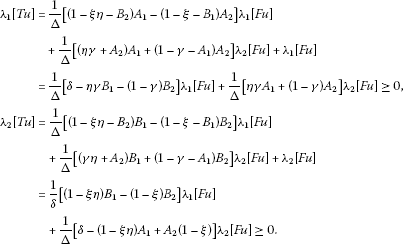Finally, we show that

$\underset{\left[0,\eta \right]}{min}Tu\left(t\right)\ge \rho {\parallel Tu\parallel }_{1}.$

To do it, we consider two steps. Let ${\parallel Tu\parallel }_{1}=Tu\left({t}^{\ast }\right)$.

Step 1. Let $Tu\left(0\right). Then ${t}^{\ast }\in \left(0,\eta \right)$ or ${t}^{\ast }\in \left(\eta ,1\right)$ and ${min}_{\left[0,\eta \right]}Tu\left(t\right)=Tu\left(0\right)$.

Let ${t}^{\ast }\in \left(0,\eta \right)$. Then

$\frac{{\parallel Tu\parallel }_{1}-Tu\left(1\right)}{Tu\left(\eta \right)-Tu\left(1\right)}\le \frac{1-{t}^{\ast }}{1-\eta },$

so

$\begin{array}{rcl}{\parallel Tu\parallel }_{1}& \le & Tu\left(1\right)+\frac{1}{1-\eta }\left[Tu\left(\eta \right)-Tu\left(1\right)\right]<\frac{1}{1-\eta }Tu\left(\eta \right)=\frac{1}{\gamma \left(1-\eta \right)}\left(Tu\left(0\right)-{\lambda }_{1}\left[u\right]\right)\\ \le & \frac{1}{\gamma \left(1-\eta \right)}Tu\left(0\right).\end{array}$

It yields

$\underset{\left[0,\eta \right]}{min}Tu\left(t\right)\ge \gamma \left(1-\eta \right){\parallel Tu\parallel }_{1}.$

Let ${t}^{\ast }\in \left(\eta ,1\right)$. Then

$\frac{{\parallel Tu\parallel }_{1}-Tu\left(0\right)}{Tu\left(\eta \right)-Tu\left(0\right)}\le \frac{{t}^{\ast }-0}{\eta -0},$

so

${\parallel Tu\parallel }_{1}\le \frac{1}{\eta }\left[Tu\left(\eta \right)+\left(\eta -1\right)Tu\left(0\right)\right]=\frac{1}{\eta }\left[\frac{1}{\gamma }\left(Tu\left(0\right)-{\lambda }_{1}\left[u\right]\right)+\left(\eta -1\right)Tu\left(0\right)\right].$

It yields

$\underset{\left[0,\eta \right]}{min}Tu\left(t\right)\ge \frac{\gamma \eta }{1+\gamma \left(\eta -1\right)}{\parallel Tu\parallel }_{1}.$

Step 2. Let $Tu\left(0\right)\ge Tu\left(\eta \right)$. Then ${t}^{\ast }\in \left(0,\eta \right)$ and ${min}_{\left[0,\eta \right]}Tu\left(t\right)=Tu\left(\eta \right)$. Then

$\frac{{\parallel Tu\parallel }_{1}-Tu\left(1\right)}{Tu\left(\eta \right)-Tu\left(1\right)}\le \frac{1-{t}^{\ast }}{1-\eta },$

so

${\parallel Tu\parallel }_{1}\le Tu\left(1\right)+\frac{1}{1-\eta }\left[Tu\left(\eta \right)-Tu\left(1\right)\right]<\frac{1}{1-\eta }Tu\left(\eta \right).$

Hence,

$\underset{\left[0,\eta \right]}{min}Tu\left(t\right)\ge \left(1-\eta \right){\parallel Tu\parallel }_{1}.$

It shows $T:K\to K$. This ends the proof. □

Remark 2 Take $dB\left(t\right)=\left(bt-1\right)\phantom{\rule{0.2em}{0ex}}dt$, $b>1$. Note that the measure changes the sign and is increasing. It is easy to show that

${B}_{1}=\frac{1}{2}\left(b-2\right),\phantom{\rule{2em}{0ex}}{B}_{2}=\frac{1}{6}\left(2b-3\right),\phantom{\rule{2em}{0ex}}{\mathcal{G}}_{2}\left(s\right)=\frac{s\left(1-s\right)}{6}\left(bs+b-3\right).$

If we assume that $b\ge 3$, then ${B}_{1}>0$, ${B}_{2}>0$, ${\mathcal{G}}_{2}\left(s\right)\ge 0$, $s\in J$.

Remark 3 Take $dA\left(t\right)=\left(a{t}^{2}-1\right)\phantom{\rule{0.2em}{0ex}}dt$, $a>1$. Note that the measure changes the sign and is increasing. It is easy to show that

${A}_{1}=\frac{1}{3}\left(a-3\right),\phantom{\rule{2em}{0ex}}{A}_{2}=\frac{1}{4}\left(a-2\right),\phantom{\rule{2em}{0ex}}{\mathcal{G}}_{1}\left(s\right)=\frac{s\left(1-s\right)}{12}\left(a{s}^{2}+as+a-6\right).$

If we assume that $a\ge 6$, then ${A}_{1}>0$, ${A}_{2}>0$, ${\mathcal{G}}_{1}\left(s\right)\ge 0$, $s\in J$.

Remark 4 Let $dA\left(t\right)=\left(3t-1\right)\phantom{\rule{0.2em}{0ex}}dt$, $dB\left(t\right)=\left(\frac{7}{2}t-1\right)\phantom{\rule{0.2em}{0ex}}dt$, $t\in J$. Then the assumptions H3, H4 hold if one of the following conditions is satisfied:

1. (i)

$\xi =0$, $0<\gamma <\frac{1}{2}$,

2. (ii)

$\gamma =0$, $0<\xi <\frac{1}{4}$,

3. (iii)

$\gamma =\xi =0$.

We consider only case (i). First of all, we see that dA, dB change the sign and are increasing. Indeed, for $p=1$, $t\in J$, we have

${A}_{1}={A}_{2}={\lambda }_{1}\left[p\right]=\frac{1}{2},\phantom{\rule{2em}{0ex}}{B}_{1}={\lambda }_{2}\left[p\right]=\frac{3}{4},\phantom{\rule{2em}{0ex}}{B}_{2}=\frac{2}{3}.$

It means that the assumption H3 holds. Moreover,It proves that the assumption H4 holds.

By a similar way, we prove the assertion in case (ii) or (iii).

## 3 Positive solutions to problem (1) with delayed arguments

Now, we present the necessary definitions from the theory of cones in Banach spaces.

Definition 1 Let E be a real Banach space. A nonempty convex closed set $P\subset E$ is said to be a cone provided that

1. (i)

$ku\in P$ for all $u\in P$ and all $k\ge 0$, and

2. (ii)

$u,-u\in P$ implies $u=0$.

Note that every cone $P\subset E$ induces an ordering in E given by $x\le y$ if $y-x\in P$.

Definition 2 A map Φ is said to be a nonnegative continuous concave functional on a cone P of a real Banach space E if $\mathrm{\Phi }:P\to {\mathbb{R}}_{+}$ is continuous and

$\mathrm{\Phi }\left(tx+\left(1-t\right)y\right)\ge t\mathrm{\Phi }\left(x\right)+\left(1-t\right)\mathrm{\Phi }\left(y\right)$

for all $x,y\in P$ and $t\in \left[0,1\right]$.

Similarly, we say the map φ is a nonnegative continuous convex functional on a cone P of a real Banach space E if $\phi :P\to {\mathbb{R}}_{+}$ is continuous and

$\phi \left(tx+\left(1-t\right)y\right)\le t\phi \left(x\right)+\left(1-t\right)\phi \left(y\right)$

for all $x,y\in P$ and $t\in \left[0,1\right]$.

Definition 3 An operator is called completely continuous if it is continuous and maps bounded sets into precompact sets.

Let φ and Θ be nonnegative continuous convex functionals on P, let Φ be a nonnegative continuous concave functional on P, and let Ψ be a nonnegative continuous functional on P. Then, for positive numbers a, b, c, d, we define the following sets: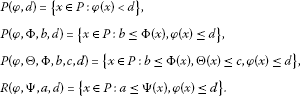We will use the following fixed point theorem of Avery and Peterson to establish multiple positive solutions to problem (1).

Theorem 1 (see )

Let P be a cone in a real Banach space E. Let φ and Θ be nonnegative continuous convex functionals on P, let Φ be a nonnegative continuous concave functional on P, and let Ψ be a nonnegative continuous functional on P satisfying $\mathrm{\Psi }\left(kx\right)\le k\mathrm{\Psi }\left(x\right)$ for $0\le k\le 1$ such that for some positive numbers $\overline{M}$ and d,

$\mathrm{\Phi }\left(x\right)\le \mathrm{\Psi }\left(x\right)\phantom{\rule{1em}{0ex}}\mathit{\text{and}}\phantom{\rule{1em}{0ex}}\parallel x\parallel \le \overline{M}\phi \left(x\right)$

for all $x\in \overline{P\left(\phi ,d\right)}$. Suppose

$T:\overline{P\left(\phi ,d\right)}\to \overline{P\left(\phi ,d\right)}$

is completely continuous and there exist positive numbers a, b, c with $a such that

(S1): $\left\{x\in P\left(\phi ,\mathrm{\Theta },\mathrm{\Phi },b,c,d\right):\mathrm{\Phi }\left(x\right)>b\right\}\ne 0$ and $\mathrm{\Phi }\left(Tx\right)>b$ for $x\in P\left(\phi ,\mathrm{\Theta },\mathrm{\Phi },b,c,d\right)$,

(S2): $\mathrm{\Phi }\left(Tx\right)>b$ for $x\in P\left(\phi ,\mathrm{\Phi },b,d\right)$ with $\mathrm{\Theta }\left(Tx\right)>c$,

(S3): $0\notin R\left(\phi ,\mathrm{\Psi },a,d\right)$ and $\mathrm{\Psi }\left(Tx\right) for $x\in R\left(\phi ,\mathrm{\Psi },a,d\right)$ with $\mathrm{\Psi }\left(x\right)=a$.

Then T has at least three fixed points ${x}_{1},{x}_{2},{x}_{3}\in \overline{P\left(\phi ,d\right)}$ such thatand

$\mathrm{\Psi }\left({x}_{3}\right)

We apply Theorem 1 with the cone K instead of P and let ${\overline{P}}_{r}=\left\{x\in K:\parallel x\parallel \le r\right\}$. Now, we define the nonnegative continuous concave functional Φ on K by

$\mathrm{\Phi }\left(x\right)=\underset{\left[0,\eta \right]}{min}|x\left(t\right)|.$

Note that $\mathrm{\Phi }\left(x\right)\le {\parallel x\parallel }_{1}$. Put $\mathrm{\Psi }\left(x\right)=\mathrm{\Theta }\left(x\right)={\parallel x\parallel }_{1},\phi \left(x\right)={\parallel {x}^{\prime }\parallel }_{1}$.

Now, we can formulate the main result of this section.

Theorem 2 Let the assumptions H1-H4 hold with $\xi =0$, $\gamma >0$. Let $\alpha \left(t\right)\le t$, $t\in J$. In addition, we assume that there exist positive constants a, b, c, d, M, $a and such that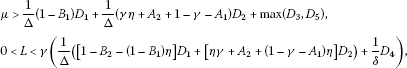with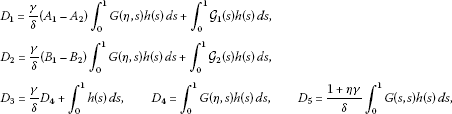and

(A1): $f\left(t,u,v\right)\le \frac{d}{\mu }$ for $\left(t,u,v\right)\in J×\left[0,Md\right]×\left[-d,d\right]$,

(A2): $f\left(t,u,v\right)\ge \frac{b}{L}$ for $\left(t,u,v\right)\in \left[0,\eta \right]×\left[b,\frac{b}{\rho }\right]×\left[-d,d\right]$,

(A3): $f\left(t,u,v\right)\le \frac{a}{\mu }$ for $\left(t,u,v\right)\in J×\left[0,a\right]×\left[-d,d\right]$.

Then problem (1) has at least three nonnegative solutions ${x}_{1}$, ${x}_{2}$, ${x}_{3}$ satisfying ${\parallel {x}_{i}^{\prime }\parallel }_{1}\le d$, $i=1,2,3$,

$b\le \mathrm{\Phi }\left({x}_{1}\right),\phantom{\rule{2em}{0ex}}a<{\parallel {x}_{2}\parallel }_{1}\phantom{\rule{1em}{0ex}}\mathit{\text{with}}\phantom{\rule{0.1em}{0ex}}\mathrm{\Phi }\left({x}_{2}\right)

and ${\parallel {x}_{3}\parallel }_{1}.

Proof Basing on the definitions of T, we see that $T\overline{P}$ is equicontinuous on J, so T is completely continuous.

Let $x\in \overline{P\left(\phi ,d\right)}$, so $\phi \left(x\right)={\parallel {x}^{\prime }\parallel }_{1}\le d$. By Lemma 1, ${\parallel x\parallel }_{1}\le Md$, so $0\le x\left(t\right)\le Md$, $t\in J$. Assumption (A1) implies $f\left(t,x\left(\alpha \left(t\right)\right),{x}^{\prime }\left(\beta \left(t\right)\right)\right)\le \frac{d}{\mu }$.

Moreover, in view of (7),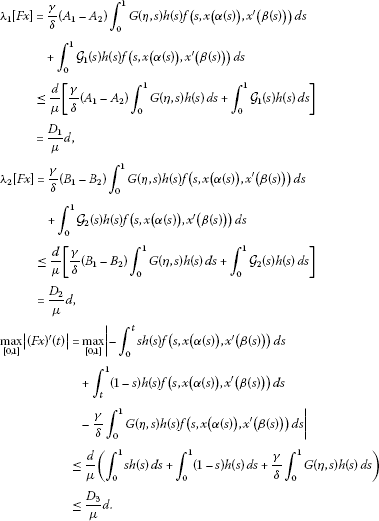Combining it, we have

$\begin{array}{rcl}\phi \left(Tx\right)& =& \underset{\left[0,1\right]}{max}|{\left(Tx\right)}^{\prime }\left(t\right)|\\ \le & \frac{1}{\mathrm{\Delta }}\left(1-{B}_{1}\right){\lambda }_{1}\left[Fx\right]+\frac{1}{\mathrm{\Delta }}\left(1-\gamma -{A}_{1}\right){\lambda }_{2}\left[Fx\right]+\underset{\left[0,1\right]}{max}|{\left(Fx\right)}^{\prime }\left(t\right)|\\ \le & \frac{d}{\mu }\left(\frac{1}{\mathrm{\Delta }}\left(1-{B}_{1}\right){D}_{1}+\frac{1}{\mathrm{\Delta }}\left(1-\gamma -{A}_{1}\right){D}_{2}+{D}_{3}\right)

This proves that $T:\overline{P\left(\phi ,d\right)}\to \overline{P\left(\phi ,d\right)}$.

Now, we need to show that condition (S1) is satisfied. Take

${x}_{0}\left(t\right)=\frac{1}{2}\left(b+\frac{b}{\rho }\right),\phantom{\rule{1em}{0ex}}t\in J.$

Then ${x}_{0}\left(t\right)>0$, $t\in J$, andfor $p\left(t\right)=1$, $t\in J$. Moreover,This proves that

$\left\{{x}_{0}\in P\left(\phi ,\mathrm{\Theta },\mathrm{\Phi },b,\frac{b}{\rho },d\right):b<\mathrm{\Phi }\left({x}_{0}\right)\right\}\ne \mathrm{\varnothing }.$

Let $b\le x\left(t\right)\le \frac{b}{\rho }$ for $t\in \left[0,\eta \right]$. Then $0\le \alpha \left(t\right)\le t\le \eta$ for $t\in \left[0,\eta \right]$, so $b\le x\left(\alpha \left(t\right)\right)\le \frac{b}{\rho }$, $t\in \left[0,\eta \right]$. Assumption (A2) implies $f\left(t,x\left(\alpha \left(t\right)\right),{x}^{\prime }\left(\beta \left(t\right)\right)\right)\ge \frac{b}{L}$. Hence,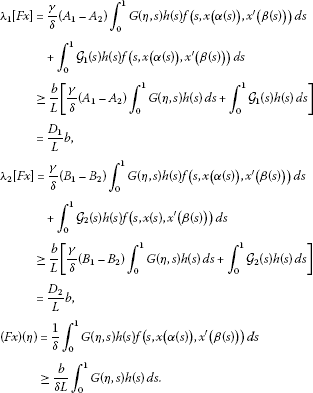Moreover,It yields

$\begin{array}{rcl}\mathrm{\Phi }\left(Tx\right)& =& \underset{\left[0,\eta \right]}{min}\left(Tx\right)\left(t\right)=min\left(\left(Tx\right)\left(0\right),\left(Tx\right)\left(\eta \right)\right)\ge \gamma \left(Tx\right)\left(\eta \right)\\ =& \frac{\gamma }{\mathrm{\Delta }}\left[1-{B}_{2}-\left(1-{B}_{1}\right)\eta \right]{\lambda }_{1}\left[Fx\right]+\frac{\gamma }{\mathrm{\Delta }}\left[\eta \gamma +{A}_{2}+\left(1-\gamma -{A}_{1}\right)\eta \right]{\lambda }_{2}\left[Fx\right]\\ +\frac{\gamma }{\delta }{\int }_{0}^{1}G\left(\eta ,s\right)h\left(s\right)f\left(s,x\left(\alpha \left(s\right)\right),{x}^{\prime }\left(\beta \left(s\right)\right)\right)\phantom{\rule{0.2em}{0ex}}ds\\ \ge & \frac{b\gamma }{L}\left(\frac{1}{\mathrm{\Delta }}\left(\left[1-{B}_{2}-\left(1-{B}_{1}\right)\eta \right]{D}_{1}+\left[\eta \gamma +{A}_{2}+\left(1-\gamma -{A}_{1}\right)\eta \right]{D}_{2}\right)+\frac{{D}_{4}}{\delta }\right)\\ >& b.\end{array}$

This proves that condition (S1) holds.

Now, we need to prove that condition (S2) is satisfied. Take $x\in P\left(\phi ,\mathrm{\Phi },b,d\right)$ and ${\parallel Tx\parallel }_{1}>\frac{b}{\rho }=c$. Then

$\mathrm{\Phi }\left(Tx\right)=\underset{\left[0,\eta \right]}{min}\left(Tx\right)\left(t\right)\ge \rho {\parallel Tx\parallel }_{1}>\rho \frac{b}{\rho }=b,$

so condition (S2) holds.

Indeed, $\phi \left(0\right)=0, so $0\notin R\left(\phi ,\mathrm{\Psi },a,d\right)$. Suppose that $x\in R\left(\phi ,\mathrm{\Psi },a,d\right)$ with $\mathrm{\Psi }\left(x\right)={\parallel x\parallel }_{1}=a$. Note that $G\left(t,s\right)\le G\left(s,s\right)$, $t\in J$. Then

$\begin{array}{rcl}{\parallel Fx\parallel }_{1}& \le & \frac{\gamma }{\delta }{\int }_{0}^{1}G\left(\eta ,s\right)h\left(s\right)f\left(s,x\left(\alpha \left(s\right)\right),{x}^{\prime }\left(\beta \left(s\right)\right)\right)\phantom{\rule{0.2em}{0ex}}ds\\ +{\int }_{0}^{1}G\left(s,s\right)h\left(s\right)f\left(s,x\left(\alpha \left(s\right)\right),{x}^{\prime }\left(\beta \left(s\right)\right)\right)\phantom{\rule{0.2em}{0ex}}ds\\ \le & \frac{a}{\mu }\left[\frac{\gamma }{\delta }{\int }_{0}^{1}G\left(\eta ,s\right)h\left(s\right)\phantom{\rule{0.2em}{0ex}}ds+{\int }_{0}^{1}G\left(s,s\right)h\left(s\right)\phantom{\rule{0.2em}{0ex}}ds\right]\\ \le & \frac{a}{\mu }{D}_{5}\end{array}$

and finally,

$\begin{array}{rcl}\mathrm{\Psi }\left(Tx\right)& =& \underset{t\in J}{max}\left(Tx\right)\left(t\right)\\ \le & \frac{1}{\mathrm{\Delta }}\left[1-{B}_{2}\right]{\lambda }_{1}\left[Fx\right]+\frac{1}{\mathrm{\Delta }}\left[\eta \gamma +{A}_{2}+1-\gamma -{A}_{1}\right]{\lambda }_{2}\left[Fx\right]+{\parallel Fx\parallel }_{1}\\ \le & \frac{a}{\mu }\left(\frac{1}{\mathrm{\Delta }}\left(\left[1-{B}_{2}\right]{D}_{1}+\left[\eta \gamma +{A}_{2}+1-\gamma -{A}_{1}\right]{D}_{2}\right)+{D}_{5}\right)\\ <& a.\end{array}$

This shows that condition (S3) is satisfied.

Since all the conditions of Theorem 1 are satisfied, problem (1) has at least three nonnegative solutions ${x}_{1}$, ${x}_{2}$, ${x}_{3}$ such that $\parallel {x}_{i}^{\prime }\parallel \le d$ for $i=1,2,3$, and

This ends the proof. □

Example Consider the following problem:

$\left\{\begin{array}{c}{x}^{″}\left(t\right)+hf\left(t,x\left(\alpha \left(t\right)\right),{x}^{\prime }\left(\beta \left(t\right)\right)\right)=0,\phantom{\rule{1em}{0ex}}t\in \left(0,1\right),\hfill \\ x\left(0\right)=\frac{1}{4}x\left(\frac{1}{2}\right),\phantom{\rule{2em}{0ex}}x\left(1\right)=\frac{1}{2}{\int }_{0}^{1}x\left(t\right)\left(7t-2\right)\phantom{\rule{0.2em}{0ex}}dt,\hfill \end{array}$
(8)

where

$f\left(t,u,v\right)=\left\{\begin{array}{cc}\frac{1}{100}cost+{\left(\frac{v}{20,000}\right)}^{2},\hfill & \left(t,u,v\right)\in \left[0,1\right]×\left[0,1\right]×\left[-d,d\right],\hfill \\ \frac{1}{100}cost+2\left(u-1\right)+{\left(\frac{v}{20,000}\right)}^{2},\hfill & \left(t,u,v\right)\in \left[0,1\right]×\left[1,16\right]×\left[-d,d\right],\hfill \\ \frac{1}{100}cost+30+{\left(\frac{v}{20,000}\right)}^{2},\hfill & \left(t,v\right)\in \left[0,1\right]×\left[-d,d\right],u\ge 16\hfill \end{array}$

with $d=2,000$. For example, we can take $\alpha \left(t\right)=\overline{\rho }t$, $\beta \left(t\right)=\sqrt{t}$ on J with fixed $\overline{\rho }\in \left(0,1\right)$. Indeed, $f\in C\left(\left[0,1\right]×{\mathbb{R}}_{+}×\left[-d,d\right],{\mathbb{R}}_{+}\right)$, $\gamma =\frac{1}{4}$, $\eta =\frac{1}{2}$, $h\left(t\right)=h>0$, $\xi =0$ and

${\lambda }_{1}\left[x\right]=0,\phantom{\rule{2em}{0ex}}{\lambda }_{2}\left[x\right]=\frac{1}{2}{\int }_{0}^{1}x\left(t\right)\left(7t-2\right)\phantom{\rule{0.2em}{0ex}}dt,\phantom{\rule{2em}{0ex}}\rho =\frac{1}{8}.$

Note that $dB\left(t\right)=\frac{1}{2}\left(7t-2\right)\phantom{\rule{0.2em}{0ex}}dt$, so the measure changes the sign on J. Moreover,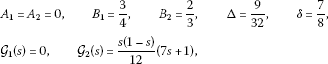so the assumption H4 holds; see Remark 4. Next,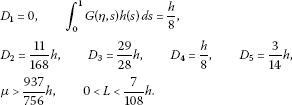Put $a=1$, $b=2$, $h=30$, then $c=16$, $\mu >37.18$, $L<1.94$. Let $\mu =40$, $L=1$. Thenand

$f\left(t,u,v\right)\le \frac{1}{100}+30+{\left(\frac{2,000}{20,000}\right)}^{2}=30.02<50=\frac{d}{\mu }$

for $\left(t,u,v\right)\in \left[0,1\right]×\left[0,2d\right]×\left[-d,d\right]$.

All the assumptions of Theorem 2 hold, so problem (8) has at least three positive solutions.

Remark 5 We can also construct an example in which, for example, ${\lambda }_{1}\left[x\right]={\int }_{0}^{1}x\left(t\right)\left(3t-1\right)\phantom{\rule{0.2em}{0ex}}dt$ to use the results of Remark 4. Note that also this measure changes the sign.

## 4 Positive solutions to problem (1) with advanced arguments

In this section, we consider the case when $\alpha \left(t\right)\ge t$ on J, so the interval $\left[0,\eta \right]$ is now replaced by $\left[\eta ,1\right]$. It means that we can put $\gamma =0$ with $\xi >0$ in the boundary conditions of problem (1) because someone can take ${\lambda }_{1}\left[x\right]=\overline{\gamma }x\left(\eta \right)$ as an example. Let us introduce the cone ${K}_{2}$ by

${K}_{2}=\left\{x\in E:x\left(t\right)\ge 0,t\in J,{\lambda }_{1}\left[x\right]\ge 0,{\lambda }_{2}\left[x\right]\ge 0,\underset{\left[\eta ,1\right]}{min}x\left(t\right)\ge \mathrm{\Gamma }{\parallel x\parallel }_{1}\right\}$

with

$\mathrm{\Gamma }=min\left(\frac{\xi \left(1-\eta \right)}{1-\xi \eta },\xi \eta ,\eta \right),\phantom{\rule{1em}{0ex}}\xi >0.$

Now $\mathrm{\Phi }\left(x\right)={min}_{\left[\eta ,1\right]}|x\left(t\right)|$. Functionals Ψ, Θ, φ are defined as in Section 3. We formulate only the main result using the cone ${K}_{2}$ instead of K (see Theorem 2); the proof is similar to the previous one.

Theorem 3 Let the assumptions H1-H4 hold with $\gamma =0$, $\xi >0$. Let $\alpha \left(t\right)\ge t$, $t\in J$. In addition, we assume that there exist positive constants a, b, c, d, M, $a and such thatwithand

(B1): $f\left(t,u,v\right)\le \frac{d}{\mu }$ for $\left(t,u,v\right)\in J×\left[0,Md\right]×\left[-d,d\right]$,

(B2): $f\left(t,u,v\right)\ge \frac{b}{L}$ for $\left(t,u,v\right)\in \left[\eta ,1\right]×\left[b,\frac{b}{\mathrm{\Gamma }}\right]×\left[-d,d\right]$,

(B3): $f\left(t,u,v\right)\le \frac{a}{\mu }$ for $\left(t,u,v\right)\in J×\left[0,a\right]×\left[-d,d\right]$.

Then problem (1) has at least three nonnegative solutions ${x}_{1}$, ${x}_{2}$, ${x}_{3}$ satisfying ${\parallel {x}_{i}^{\prime }\parallel }_{1}\le d$, $i=1,2,3$,

$b\le \mathrm{\Phi }\left({x}_{1}\right),\phantom{\rule{2em}{0ex}}a<{\parallel {x}_{2}\parallel }_{1}\phantom{\rule{1em}{0ex}}\mathit{\text{with}}\phantom{\rule{0.1em}{0ex}}\mathrm{\Phi }\left({x}_{2}\right)

and ${\parallel {x}_{3}\parallel }_{1}.

## 5 Positive solutions to problem (1) for the case when $\alpha \left(t\right)=t$on J

In this section, we consider problem (1) when $\alpha \left(t\right)=t$ on J and $\gamma =\xi =0$. It means that now $\mathrm{\Phi }\left(x\right)={min}_{\left[\zeta ,\varrho \right]}|x\left(t\right)|$ for some fixed constants ζ, ϱ such that $0<\zeta <\varrho <1$. For $0<\zeta +\varrho <1$ we can show that $G\left(t,s\right)\ge \kappa G\left(s,s\right)$, $t\in \left[\zeta ,\varrho \right]$, $s\in J$. Now, for $\kappa =min\left(\zeta ,1-\varrho \right)$, we introduce the cone ${K}_{3}$ by

${K}_{3}=\left\{x\in E:x\left(t\right)\ge 0,t\in J,{\lambda }_{1}\left[x\right]\ge 0,{\lambda }_{2}\left[x\right]\ge 0,\underset{\left[\zeta ,\varrho \right]}{min}x\left(t\right)\ge \kappa {\parallel x\parallel }_{1}\right\}.$

Functionals Ψ, Θ, φ are defined as in Section 3; the cone K is now replaced by ${K}_{3}$.

Theorem 4 Let the assumptions H1-H4 hold with $\gamma =\xi =0$. Let $0<\zeta +\varrho <1$, $\alpha \left(t\right)=t$, $t\in J$. In addition, we assume that there exist positive constants a, b, c, d, M, $a and such that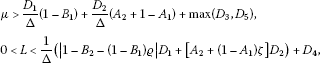with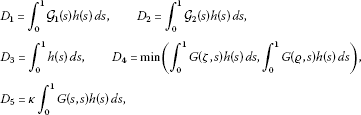and

(C1): $f\left(t,u,v\right)\le \frac{d}{\mu }$ for $\left(t,u,v\right)\in J×\left[0,Md\right]×\left[-d,d\right]$,

(C2): $f\left(t,u,v\right)\ge \frac{b}{L}$ for $\left(t,u,v\right)\in \left[\zeta ,\varrho \right]×\left[b,\frac{b}{\kappa }\right]×\left[-d,d\right]$,

(C3): $f\left(t,u,v\right)\le \frac{a}{\mu }$ for $\left(t,u,v\right)\in J×\left[0,a\right]×\left[-d,d\right]$.

Then problem (1) has at least three nonnegative solutions ${x}_{1}$, ${x}_{2}$, ${x}_{3}$ satisfying ${\parallel {x}_{i}^{\prime }\parallel }_{1}\le d$, $i=1,2,3$,

$b\le \mathrm{\Phi }\left({x}_{1}\right),\phantom{\rule{2em}{0ex}}a<{\parallel {x}_{2}\parallel }_{1}\phantom{\rule{1em}{0ex}}\mathit{\text{with}}\phantom{\rule{0.1em}{0ex}}\mathrm{\Phi }\left({x}_{2}\right)

and ${\parallel {x}_{3}\parallel }_{1}.

## 6 Conclusions

In this paper, we have discussed boundary value problems for second-order differential equations with deviating arguments and with dependence on the first-order derivative. In our research, the deviating arguments can be both delayed and advanced. By using the fixed point theorem of Avery and Peterson, new sufficient conditions for the existence of positive solutions to such boundary problems have been derived. An example is provided for illustration.

## References

1. Avery RI, Peterson AC: Three positive fixed points of nonlinear operators on ordered Banach spaces. Comput. Math. Appl. 2001, 42: 313-322. 10.1016/S0898-1221(01)00156-0

2. Bai Z, Ge W: Existence of three positive solutions for some second-order boundary value problems. Comput. Math. Appl. 2004, 48: 699-707. 10.1016/j.camwa.2004.03.002

3. Graef JR, Webb JRL: Third order boundary value problems with nonlocal boundary conditions. Nonlinear Anal. 2009, 71: 1542-1551. 10.1016/j.na.2008.12.047

4. Guo Y, Ge W: Positive solutions for three-point boundary value problems with dependence on the first order derivative. J. Math. Anal. Appl. 2004, 290: 291-301. 10.1016/j.jmaa.2003.09.061

5. Guo Y, Yu C, Wang J: Existence of three positive solutions for m -point boundary value problems on infinite intervals. Nonlinear Anal. 2009, 71: 717-722. 10.1016/j.na.2008.10.126

6. Infante G, Pietramala P: Nonlocal impulsive boundary value problems with solutions that change sign. In Mathematical Models in Engineering, Biology and Medicine. Proceedings of the International Conference on Boundary Value Problems Edited by: Cabada A, Liz E, Nieto JJ. 2009, 205-213.

7. Infante G, Pietramala P, Zima M: Positive solutions for a class of nonlocal impulsive BVPs via fixed point index. Topol. Methods Nonlinear Anal. 2010, 36: 263-284.

8. Jankowski T: Positive solutions to second order four-point boundary value problems for impulsive differential equations. Appl. Math. Comput. 2008, 202: 550-561. 10.1016/j.amc.2008.02.040

9. Jankowski T: Positive solutions of three-point boundary value problems for second order impulsive differential equations with advanced arguments. Appl. Math. Comput. 2008, 197: 179-189. 10.1016/j.amc.2007.07.081

10. Jankowski T: Existence of positive solutions to second order four-point impulsive differential equations with deviating arguments. Comput. Math. Appl. 2009, 58: 805-817. 10.1016/j.camwa.2009.06.001

11. Jankowski T: Three positive solutions to second-order three-point impulsive differential equations with deviating arguments. Int. J. Comput. Math. 2010, 87: 215-225. 10.1080/00207160801994149

12. Jankowski T: Multiple solutions for a class of boundary value problems with deviating arguments and integral boundary conditions. Dyn. Syst. Appl. 2010, 19: 179-188.

13. Jankowski T: Positive solutions for second order impulsive differential equations involving Stieltjes integral conditions. Nonlinear Anal. 2011, 74: 3775-3785. 10.1016/j.na.2011.03.022

14. Jankowski T: Existence of positive solutions to third order differential equations with advanced arguments and nonlocal boundary conditions. Nonlinear Anal. 2012, 75: 913-923. 10.1016/j.na.2011.09.025

15. Ji D, Ge W: Multiple positive solutions for some p -Laplacian boundary value problems. Appl. Math. Comput. 2007, 187: 1315-1325. 10.1016/j.amc.2006.09.041

16. Ji D, Ge W: Existence of multiple positive solutions for one-dimensional p -Laplacian operator. J. Appl. Math. Comput. 2008, 26: 451-463. 10.1007/s12190-007-0030-3

17. Karakostos GL, Tsamatos PC: Existence of multipoint positive solutions for a nonlocal boundary value problem. Topol. Methods Nonlinear Anal. 2002, 19: 109-121.

18. Sun B, Qu Y, Ge W: Existence and iteration of positive solutions for a multipoint one-dimensional p -Laplacian boundary value problem. Appl. Math. Comput. 2008, 197: 389-398. 10.1016/j.amc.2007.07.071

19. Sun B, Ge W, Zhao D: Three positive solutions for multipoint one-dimensional p -Laplacian boundary value problems with dependence on the first order derivative. Math. Comput. Model. 2007, 45: 1170-1178. 10.1016/j.mcm.2006.10.002

20. Wang W, Sheng J: Positive solutions to a multi-point boundary value problem with delay. Appl. Math. Comput. 2007, 188: 96-102. 10.1016/j.amc.2006.09.093

21. Wang Y, Ge W: Multiple positive solutions for multipoint boundary value problems with one-dimensional p -Laplacian. J. Math. Anal. Appl. 2007, 327: 1381-1395. 10.1016/j.jmaa.2006.05.023

22. Wang Y, Ge W: Existence of triple positive solutions for multi-point boundary value problems with one-dimensional p -Laplacian. Comput. Math. Appl. 2007, 54: 793-807. 10.1016/j.camwa.2006.10.038

23. Wang Y, Zhao W, Ge W: Multiple positive solutions for boundary value problems of second order delay differential equations with one-dimensional p -Laplacian. J. Math. Anal. Appl. 2007, 326: 641-654. 10.1016/j.jmaa.2006.03.028

24. Wang Z, Zhang J: Positive solutions for one-dimensional p -Laplacian boundary value problems with dependence on the first order derivative. J. Math. Anal. Appl. 2006, 314: 618-630. 10.1016/j.jmaa.2005.04.012

25. Webb JRL, Infante G: Positive solutions of nonlocal boundary value problems: a unified approach. J. Lond. Math. Soc. 2006, 74: 673-693. 10.1112/S0024610706023179

26. Webb JRL, Infante G: Positive solutions of nonlocal boundary value problems involving integral conditions. Nonlinear Differ. Equ. Appl. 2008, 15: 45-67. 10.1007/s00030-007-4067-7

27. Webb JRL, Infante G: Non-local boundary value problems of arbitrary order. J. Lond. Math. Soc. 2009, 79: 238-259.

28. Yan B, O’Regan D, Agarwal RP: Multiple positive solutions of singular second order boundary value problems with derivative dependence. Aequ. Math. 2007, 74: 62-89. 10.1007/s00010-006-2850-x

29. Yang L, Liu X, Jia M: Multiplicity results for second-order m -point boundary value problem. J. Math. Anal. Appl. 2006, 324: 532-542. 10.1016/j.jmaa.2005.07.084

30. Yang C, Zhai C, Yan J: Positive solutions of the three-point boundary value problem for second order differential equations with an advanced argument. Nonlinear Anal. 2006, 65: 2013-2023. 10.1016/j.na.2005.11.003

31. Yang Y, Xiao D: On existence of multiple positive solutions for ϕ -Laplacian multipoint boundary value. Nonlinear Anal. 2009, 71: 4158-4166. 10.1016/j.na.2009.02.080

## Author information

Authors

### Competing interests

The author declares that he has no competing interests.

## Rights and permissions

Reprints and Permissions

Jankowski, T. Positive solutions to second-order differential equations with dependence on the first-order derivative and nonlocal boundary conditions. Bound Value Probl 2013, 8 (2013). https://doi.org/10.1186/1687-2770-2013-8

• Accepted:

• Published:

• DOI: https://doi.org/10.1186/1687-2770-2013-8

### Keywords

• boundary value problems with delayed and advanced arguments
• nonlocal boundary conditions
• cone
• existence of positive solutions
• a fixed point theorem